# Newton's Third Law

Idea: Forces in nature always exist in pairs. Newton's third law states: For every action, there is an equal and opposite reaction. When two bodies interact: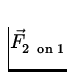= -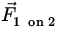. (4)
Whereis the force exerted on body 1 by body 2 and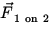is the force exerted on body 2 by body 1.

For Example: When an object falls towards the earth, the earth exerts a force on it that causes it to accelerate towards the earth. According to Newton's third law, the object exerts a force on the earth as well, and the earth accelerates towards the object. Why don't we feel the earth accelerate?
Solution:

 2nd Law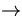meae =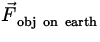3rd Law= -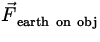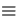-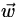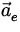= -/me|| =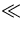g.
Conclusion: the acceleration of the earth is too small to detect because the mass of the earth is much larger than the mass of the object.Next: Applications of Newton's Laws Up: Force Previous: Newton's Second Law